# Day 45(DL) — Weights initialization and Minibatches in NN

Before moving on to the Neural Nets used for Image Classification, it is essential to understand the concepts such as Batch Normalization, Minibatches and Weight initialisation. The name ‘Batch Normalization’ seems to be very familiar. Yes, it is the same standard scaler(zscore) we have used in the Machine Learning for data normalisation. But how do we use it in Deep Learning? Is it sufficient to scale only the input data? What do we do with the output of each hidden layer? (batch normalization will be discussed in Day47).

Table of contents:

• Weights & bias Initialisation
• Minibatches in the neural networks

Weights & bias Initialisation: Previously, when we implemented a binary classifier using neural networks, the weights were initialised to random numbers and then multiplied with 0.01. Why random, we could have simply given the starting values as zero or some constant to all the weights and bias.

• The reason being, if all the learnable parameters carry similar value, then there will not be effective learning at all. Since all the neurons will try to learn similar information which completely breaks the objective of the Deep Learning itself. In order to give the ability to each neuron to acquire something new, the randoms weights are taken to kick start the learning process. This approach is also called breaking symmetry(making every neuron distinctive).
• What if the weights initialized are either too small or too large?. When the weights carry a very less magnitude, then the gradient descent will take tiny steps trying to reach the minima(as the derivative of the loss function is taken with respect to the learnable parameters). In such circumstances, the learning will be too slow resulting in not even reaching the minima. This is also termed as vanishing gradient problem. In other words, when the gradient is backpropagated through the layers, it will not completely reach all the layers(especially the initial ones).
• On the other hand, if the parameters are assigned with huge values, then the gradient descent will take gigantic steps crossing the minima without actually touching it. Here also, we failed to meet the objective of reaching the minima. This comes under the category of exploding gradient.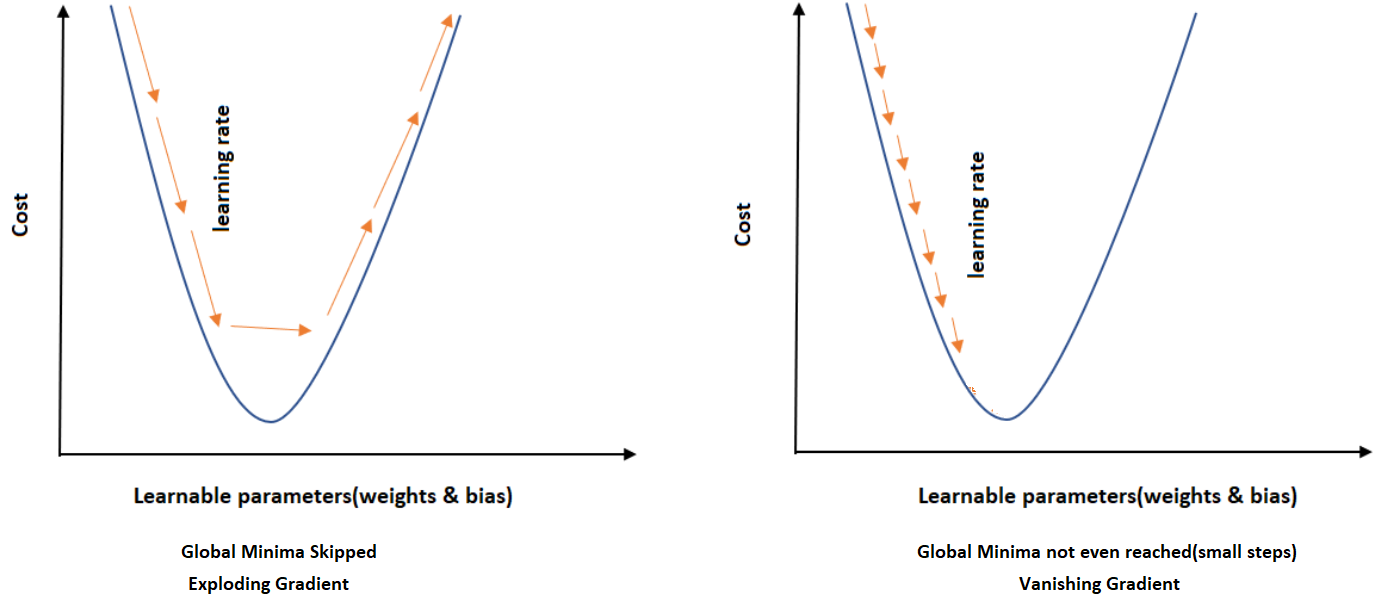Fig 1 — shows Exploding & Vanishing Gradients
• The appropriate initialization is Xavier(in general) and He for ‘ReLU’. We can refer to the recommended links for the formula derivation of why it is used along with some great visuals. So the common choice would be Xavier initialization. Since half of the values in the ‘ReLU’ activation function is zero, we can consider the same equation by dividing the neuron count by 2.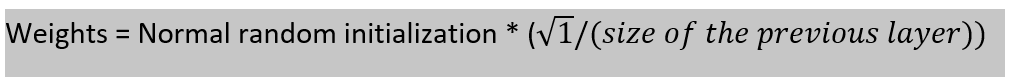The formula for Xavier initialization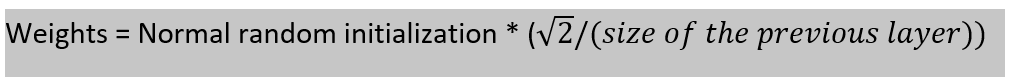The formula for He initialization

Minibatches in the neural networks: The process flow of the neural network is as follows,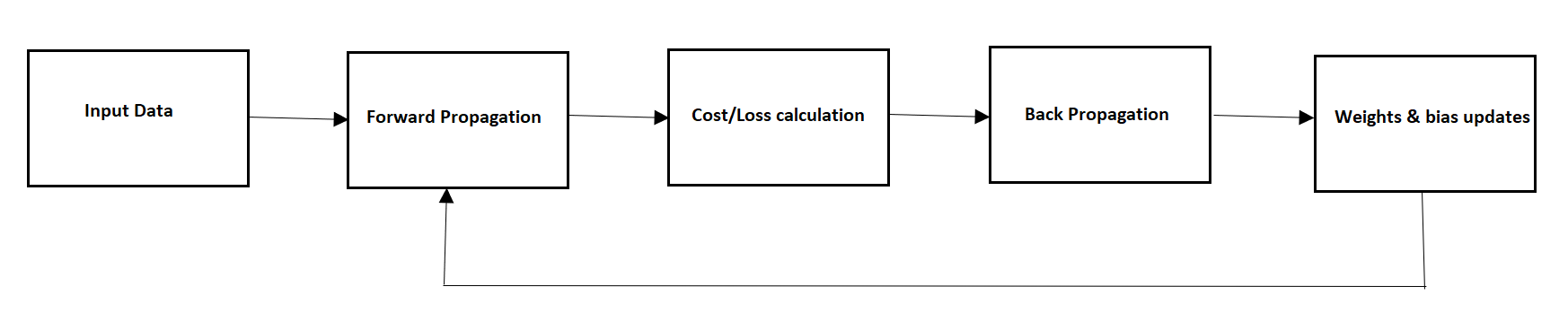Fig 2 — shows the process flow of neural networks
• In order to make a single update to the parameters, the entire training batch has to undergo the complete process. Since the objective is to optimize the parameters(weights & bias) for the global minima, one gradient descent step requires the data to be fully looped. This will result in heavy computational cost along with huge time consumption.
• An effective method to overcome the shortcomings is by introducing mini-batches. The data is split into ’n’ number of mini-batches. For every batch, the process flow happens so as the update to the learnable parameters. As a consequence, the model reaches minima sooner. But it does come with the cost of missing the smooth movement in the right direction. Let’s check that with a picture. When the data is segmented, each portion tries to modify the direction as per the information contained within. And for the very same reason, there is a small loss in direction.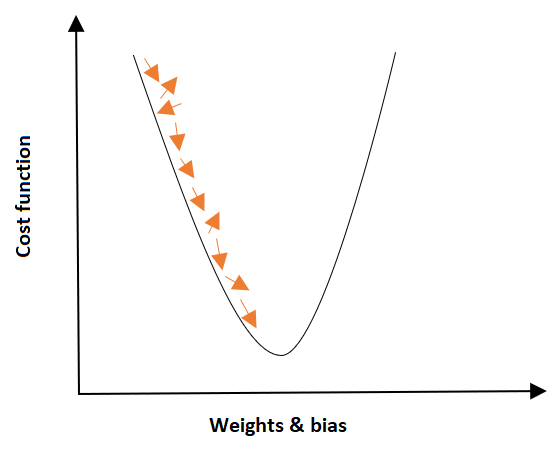Fig3 — shows the path is zigzag and not smooth
• Even though here the penalty(deviation) is issued, this approach is comparatively superior to the entire batch process. We have different optimization techniques to speed up the travel(will be discussed in the next set of articles).

Notes: In deep learning, one epoch corresponds to the entire training set. In spite of splitting the data into batches, the concept of epoch still holds true(it has to iterate through the ’n’ batches to complete an epoch).

Recommended Reading:

https://arxiv.org/abs/1502.01852v1